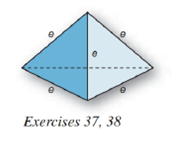Chapter 9.2, Problem 37EElementary Geometry For College St...

7th Edition
Alexander + 2 others
ISBN: 9781337614085

Solutions

Chapter
SectionElementary Geometry For College St...

7th Edition
Alexander + 2 others
ISBN: 9781337614085
Textbook Problem

A regular tetrahedron is a regular triangular pyramid in which all faces (lateral faces and base) are congruent. If each edge has length e ,a) show that the area of each face is A = e 2 3 4 .b) show that the total area of the tetrahedron is T = e 2 3 .c) find the total area if each side measures e = 4 i n .To determine

(a)

To show:

The area of each face is A=e234.

Explanation

Given:

A regular triangular pyramid in which all faces (lateral faces and base) are congruent and each edge has length e as below,

Properties Used:

The lateral area L of a regular pyramid with slant height of length l and perimeter P of the base is given by

L=12lP.

In a regular pyramid, the lengths of the apothem a of the base, the altitude h, and the slant height l satisfy the Pythagorean Theorem; that is, l2=a2+h2.

According to the Pythagorean theorem, in a right-angled triangle hypotenuse2=base2+perpendicular2.

Area of a right-angled triangle is 12×base×height.

Approach Used:

i) Using the property l2=a2+h2, find the height or altitude h by substituting the values of l and a.

ii) Substitute the values of base and height h in equation 12×base×height to get the area of each lateral face since it is a triangular pyramid.

iii) Compare the given value with the value from step (ii).

Calculation:

From the given figure

the sides of the square base and lateral edge all are e.

Considering the right-angled triangle with triangle base and slant height e

To determine

(b)

To show:

The total area of the tetrahedron is T=e23.

To determine

(c)

To find:

The total area of the tetrahedron.

Still sussing out bartleby?

Check out a sample textbook solution.

See a sample solution

The Solution to Your Study Problems

Bartleby provides explanations to thousands of textbook problems written by our experts, many with advanced degrees!

Get Started